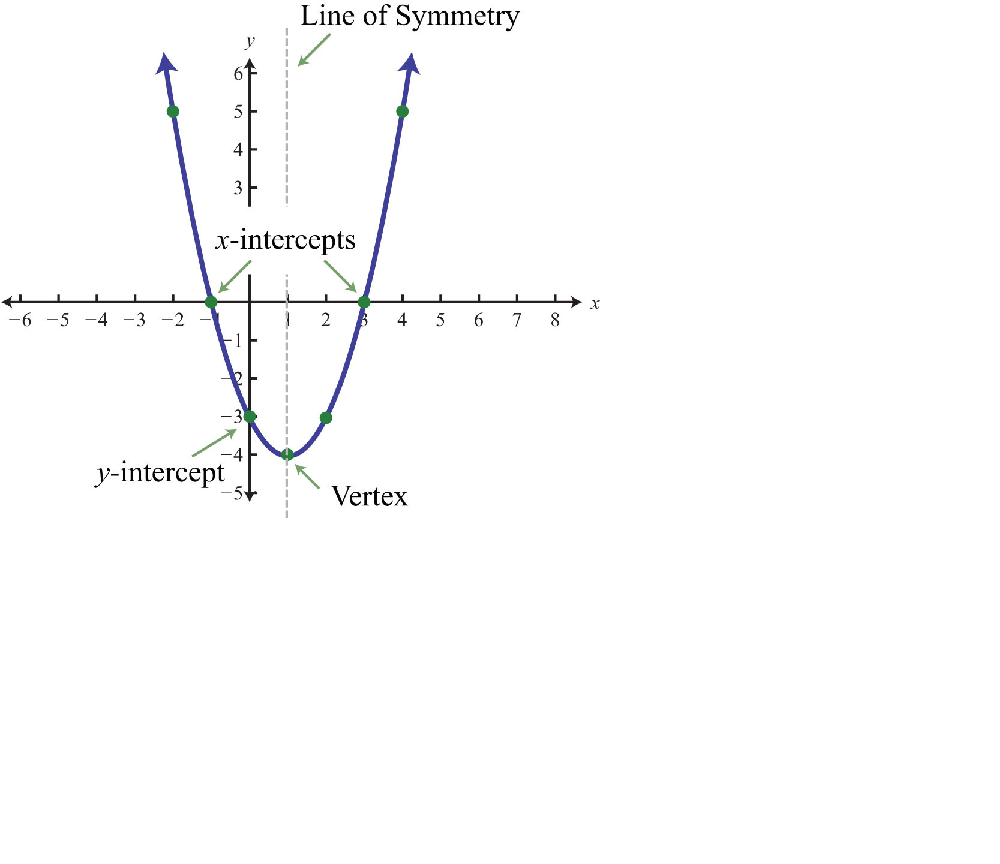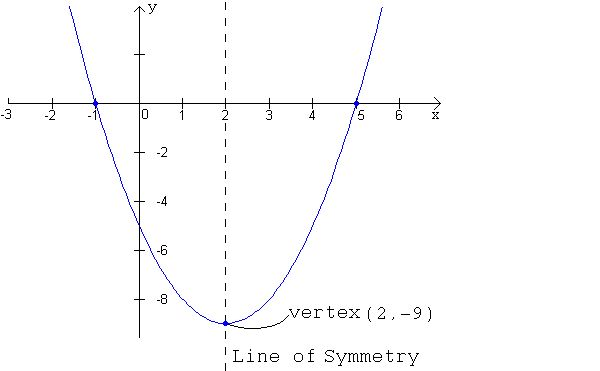# Parabola Intercept Form: Definition & Explanation

An error occurred trying to load this video.

Try refreshing the page, or contact customer support.

Coming up next: What is Prime Factorization? - Definition & Examples

### You're on a roll. Keep up the good work!

Replay
Your next lesson will play in 10 seconds
• 0:00 What Is a Parabola?
• 1:24 Everyday Uses of a Parabola
• 1:40 Intercept Form of a Parabola
• 2:23 Examples
• 3:15 Lesson Summary

Want to watch this again later?

Timeline
Autoplay
Autoplay
Speed

#### Recommended Lessons and Courses for You

Lesson Transcript
Instructor: Sarah Spitzig

Sarah has taught secondary math and English in three states, and is currently living and working in Ontario, Canada. She has recently earned a Master's degree.

In this lesson, we will define a parabola, discover its everyday uses, determine the functions of the intercept form of the equation, and find the x-intercepts of a parabola.

## What Is a Parabola?

Before we can understand parabola intercept form, we first need to go through a few other definitions, starting with a parabola. A parabola is a U-shaped graph that always has an x-squared term in its equation. The graph will open either up, like a smiley face, or down, like a sad face, and the vertex will be the lowest point if it opens up and the highest point if it opens down. In this graph, the vertex is (1,-4) and is the lowest point. When the vertex is the lowest point, it's called a minimum, and when it's the highest point, it's called a maximum.The next definition we need to know about is the line of symmetry. The line of symmetry is a vertical line that passes through the vertex and cuts the parabola in half. Any image on one side of the parabola has a mirror image on the other side of the line of symmetry. The x-intercepts are always equidistant from the line of symmetry. Going back to our previous graph, you can see the line of symmetry is x = 1.

Next, you should have an understanding of the x- and y-intercepts. The y-intercept is the point where the graph passes through the y-axis. In our graph, the y-intercept is (0, -3). The x-intercepts are where the graph passes through the x-axis. In our graph, the x-intercepts are (-1, 0) and (3, 0).

## Everyday Uses of a Parabola

The graph of a parabola has many uses in the real world. It can approximate the path of a projectile, such as a ball, plane, or rocket. It can also demonstrate the shape of a bridge, roller coaster, arch, or even the path of water in a drinking fountain.

## Intercept Form of a Parabola

There are many forms of the parabola, such as vertex form, standard form, and intercept form. Each form has special qualities that help tell us specific things about the graph. For example, vertex form helps us determine the vertex by looking at the equation, standard form helps us see the y-intercept without graphing, and intercept form helps us find the x-intercepts of the graph without factoring or using the quadratic formula.

The intercept form is y = a(x - r)(x - s), where r and s are the x-intercepts on the graph. The intercept form will tell us if there are two x-intercepts, one x-intercept or no x-intercepts.

## Examples

Let's go over some examples.

Look at the graph of the equation y = 1(x + 1)(x - 5).To unlock this lesson you must be a Study.com Member.

### Register to view this lesson

Are you a student or a teacher?

### Unlock Your Education

#### See for yourself why 30 million people use Study.com

##### Become a Study.com member and start learning now.
Back
What teachers are saying about Study.com

### Earning College Credit

Did you know… We have over 160 college courses that prepare you to earn credit by exam that is accepted by over 1,500 colleges and universities. You can test out of the first two years of college and save thousands off your degree. Anyone can earn credit-by-exam regardless of age or education level.